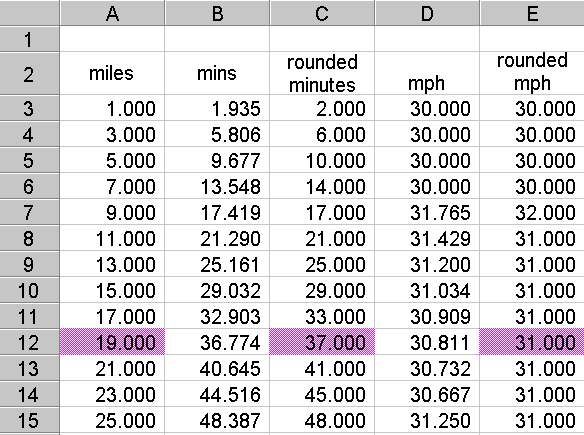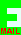## PRIME JOURNEY

This puzzle is designed for solving with a spreadsheet. Other ways are possible, of course, but it makes a good spreadsheet exercise.

A man travels a prime number of miles in a prime number of minutes at an average speed of 31 miles per hour (which is another prime number).

Now that is not possible if all the figures are exact. Additional information is that the figure for the miles travelled is exact and the number of minutes is also exact, and both are prime numbers. The figure for the average speed, however, is a rounded number; i.e. the actual speed has been rounded to the nearest whole number, which is 31.

What is the smallest number of miles for the length of the journey?

## ANSWER, and method of solution

Scroll down.

The answer is 19 miles in 37 minutes.

You can see a spreadsheet diagram by scrolling down further but you can construct it yourself as follows:

In column 1 make a series of odd numbers to represent miles . To do this type "1" in cell A3 and use the Fill Down Series in intervals of 2. This list includes all the odd primes. The number two is also a prime number but is not the answer because 2 miles at 31 mph is approximately 4 minutes which is not a prime number. Type the heading "miles" in cell A2.

In cell B2 type the heading "mins". In cell B3 compute the minutes using the formula "=A3/31*60". Fill Down from this entry to the same length as column 1. This gives a guide to the number of minutes, although what we really need is a series of whole numbers. So..

In cell C2 type the heading "rounded mins" and in cell C3 type the formula "=round(B3,0)". Fill Down as before. These are the whole number minutes we will use.

Next we need to see the speed using the rounded minutes. Type the heading "mph" in cell D2 and in D3 type the formula which is "=A3/C3*60". Fill down as before. This column will start off with the value 30 but not all the numbers will be integers.

We now need to see the rounded values of mph. Type the heading "rounded mph" in cell E2 and the formula "=round(c3,0)" in cell E3. Fill Down this column and you have the complete table.

All you have to do now is to inspect the table for the required prime miles, minutes and "31" for the rounded mph. If your table is like mine you will find the answer on line 12.

Here is a screen image I have made. All the numbers have been set to "fixed" with three decimal places.If you have any questions or problems about this, please email me, David Broughton. My email address can be obtained by clicking this symbol.

## Who got it right?

I received 4 correct answers and one wrong one. The correct answers were from John Stafford, Len Brent, Colin Rowe and John Rackett. John Racket won the £5 book token in the draw. The wrong answer was 41 miles in 79 minutes which was the next pair of prime miles and minutes in the table, but the question asked for the smallest. Thank you for all your answers.

[home] [back]